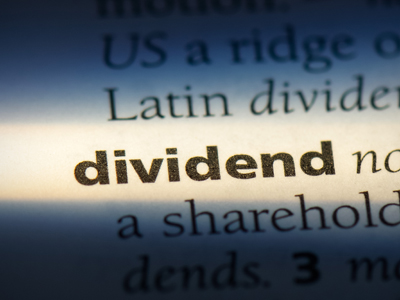Do you know the meaning of the word, 'dividend'?

# Following Instructions 3

This is the third in our Eleven Plus Following Instructions Verbal Reasoning quizzes. By now you will be very familiar with these kinds of questions, and also with the terms you need to know. Nevertheless, it never hurts to have things explained, so let’s look at terms to do with division.

• The quotient is the answer you will get when you divide a number by another: in 8 ÷ 2 = 4, the quotient is 4
• The dividend is a number which is divided by another: in 8 ÷ 2 = 4, the dividend is 8
• The divisor is a number which another number is divided by: in 8 ÷ 2 = 4, the divisor is 2

I hope that has cleared things up. Many people struggle to remember these terms – make sure you are not one of them! And now on to the quiz. Good luck!

Example:
Multiply the first figure by the sum of the last two:
8, 5, 4

The sum of the last two digits is 9 (5 + 4 = 9). The answer is 9 x 8 = 72
1.
Multiply the first figure by the sum of the last two:
12, 8, 13
1,248
144
252
248
The sum of the last two digits is 21 (8 + 13 = 21). The answer is 12 x 21 = 252
2.
Subtract the first figure from the second, then multiply your answer by the third:
27, 39, 12
132
-144
-132
144
The second figure minus the first is 12 (39 - 27 = 12). The answer is 12 x 12 = 144
3.
Multiply the second figure by the sum of the first and last:
8, 11, 3
264
122
242
121
The sum of the first and last figures is 11 (8 + 3 = 11). The answer is 11 x 11 = 121
4.
Add the last figure to the product of the first two:
3, 14, 12
29
56
36
54
The product of the first two figures is 42 (3 x 14 = 42). The answer is 42 + 12 = 54
5.
Add the first figure to the product of the last two:
34, 6, 8
82
48
56
74
The product of the last two digits is 48 (6 x 8 = 48). The answer is 34 + 48 = 82
6.
Subtract the first figure from the quotient of the last two (the last figure is the dividend and the middle one is the divisor):
17, 8, 96
5
-5
-4
-3
The quotient of the last two figures is 12, if 96 is the dividend and 8 the divisor (96 ÷ 8 = 12). The answer is 12 - 17 = -5
7.
Subtract the first figure from the product of the last two:
52, 4, 23
40
-25
30
50
The product of the last two figures is 92 (4 x 23 = 92). The answer is 92 - 52 = 40
8.
Multiply the last figure by the sum of the first two:
9, 8, 9
81
648
153
468
The sum of the first two figures is 17 (9 + 8 = 17). The answer is 9 x 17 = 153
9.
Add the first figure to the quotient of the last two (the middle figure is the dividend and the last is the divisor):
18, 36, 3
30
31
29
32
The quotient of the last two figures is 12, if 36 is the dividend and 3 the divisor (36 ÷ 3 = 12). The answer is 18 + 12 = 30
10.
Subtract the third figure from the quotient of the first two (the first figure is the dividend and the second is the divisor):
81, 9, 5
5
4
-4
-5
The quotient of the first two figures is 9, if 81 is the dividend and 9 the divisor (81 ÷ 9 = 9). The answer is 9 - 5 = 4
Author:  Stephen O'Hara and Ian Miles